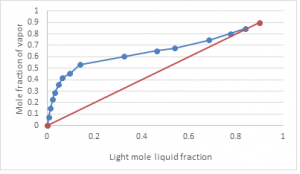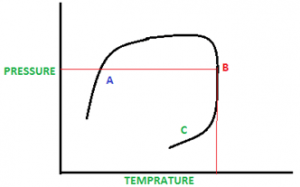Q.1
• a) Error
• b) Shift
• c) Dynamic
• d) Static
Q.2
Q.3
• a) Feed
• b) Reflux
• c) Trays
• d) Stages
Q.4
##### A reversed temperature profile, means problem with
• a) Column temperature
• b) Stage temperature
• c) Feed temperature
• d) Plate temperature
Q.5
• a) H value
• b) S value
• c) T value
• d) K value
Q.6
##### Aij is the?
• a) Absorption factor
• b) Mass transfer area
• c) Activity of i and j
• d) Stripping Factor
Q.7
##### C is the?
• a) Heat capacity
• b) Coefficient
• c) Total component
• d) Final component
Q.8
##### For the below graph the Pinch point is at?
• a) 5th stage
• b) 9th stage
• c) 10th stage
• d) 2nd stage
Q.9
##### Bubble point is at?• a) A
• b) B
• c) C
• d) Wrong interpretation
Q.10
##### In the same diagram, Critical point is at?• a) A
• b) B
• c) C
• d) Wrong interpretation